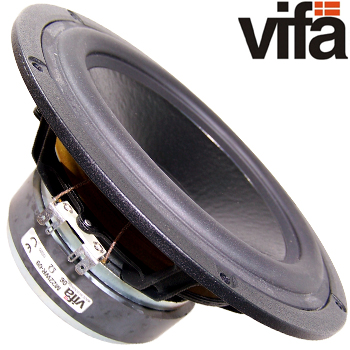# Vifa M22WR-09 6 ohm Woofer - DISCONTINUEDReplacement for Apogee slant 8 Woofer

A 6 Ohm 8" woofer with a long stroke, low reflection, magnesium basket. Very stiff conical paper cone, low dampping rubber surround and 2" voice coil.

Specification:

• Nominal Impedance = 6 ohms
• Voice Coil Resistance = 4.3 ohms
• Nominal Power (IEC268-5) = 150W
• Music Power (DIN45500) = 200W
• Operating Power = 7.5W
• Sensitivity (1W,1m) = 88dB
• Frequency Range = 30-1000 Hz
• Free Air Resonance = 30 Hz
• Voice Coil Diameter = 50mm
• Voice Coil Height = 21mm
• Air Gap Height = 8mm
• Voice Coil Inductance = 1.1 mH
• Effective Cone Area = 224cm sq
• Moving Mass (incl air) = 36g
• Magnet Weight (37.4oz)=1062g
• Force Factor, B X L = 8.8Txm
• Vas = 55 ltr
• Qms = 2.73
• Qes = 0.38
• Qts = 0.33

Price: (1off)
£74.90 +vat +p&p

Big Picture
PDF datasheet